What does it do?

Searches for a value in the first row of a table array and returns a value in the same column from another row (downwards) in the table array.

Formula breakdown:

=HLOOKUP(lookup_value, table_array, row_index_num, [range_lookup])

What it means:

=HLOOKUP(this value, in this list, and get me value in this row, [Exact Match/FALSE/0])

Ever had a horizontal table and you want to search for values in the table easily?

I’m sure you do!  There is a simple way to do this with Excel’s HLOOKUP function!

This is very similar to the VLOOKUP Function! The only difference is instead of working with vertical tables, you get to do the same thing for horizontal tables!

Let’s try it out on this horizontal table!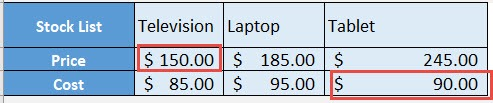Using the HLOOKUP function let us get the following values from this table:

• What is the price of a television?
• What is the cost of a tablet?

I explain how you can do this below:

STEP 1: Let us target the first question: What is the price of a television?

We need to enter the HLOOKUP function in a blank cell:

## =HLOOKUP(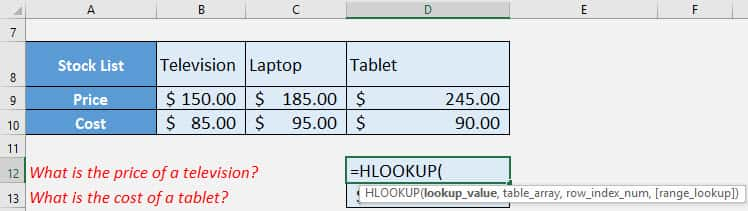STEP 2: The HLOOKUP arguments:

## lookup_value

What is the name of the column?

We want to find the column that matches “Television”

## =HLOOKUP(“Television”,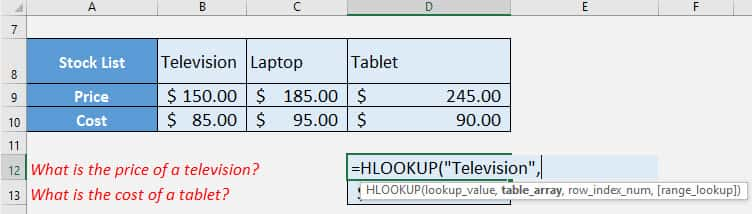## table_array

What is our list?

Select the entire table!

## =HLOOKUP(“Television”, A8:D10,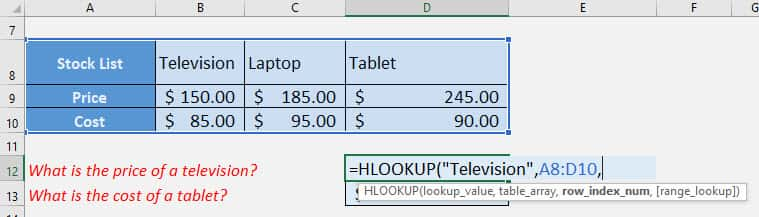## row_index_num

Which row should we get our value from?

We want the price, so it’s row #2 in our table!

## =HLOOKUP(“Television”, A8:D10, 2,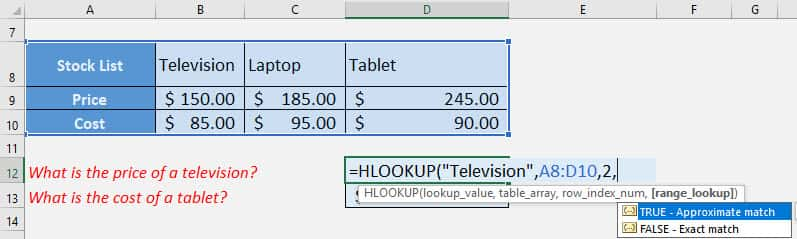## [range_lookup]

Do we want an appropriate match or exact match?

We want an exact match, so specify FALSE here.

## =HLOOKUP(“Television”, A8:D10, 2, FALSE)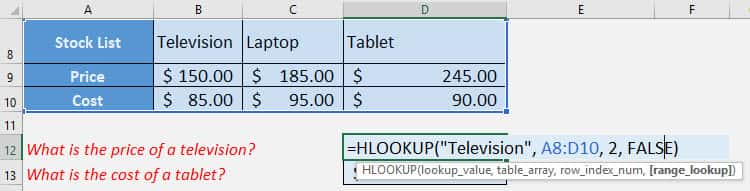You now have your television price!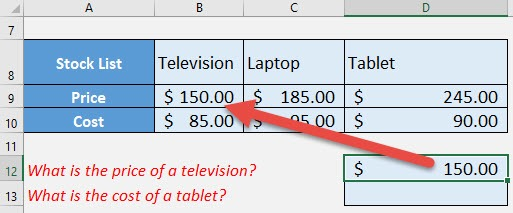STEP 3: Now let us try doing the same for the cost of the Tablet!

The column name is “Tablet”, and the cost is on row #3 in our table:

## =HLOOKUP(“Tablet”, A8:D10, 3, FALSE)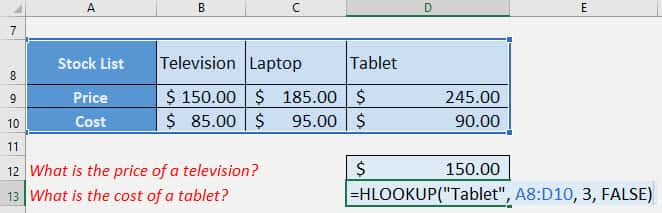You now have your tablet cost!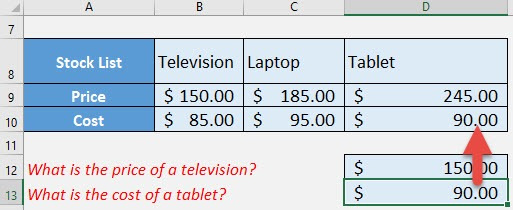HLOOKUP Function in Excel